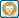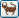Join the forum, it's quick and easy

Would you like to react to this message? Create an account in a few clicks or log in to continue.

# [分享]快速刪除物件(LISP)

+2
Tiger&蘋果爸
devinchou
6 posters##[分享]快速刪除物件(LISP)

`　;　[AutoCAD EXTEND] QUICK ERASE(BY LAYER ERASE OBJECT).　;　[AutoCAD "指令"功能延伸] 快速刪除(依圖層刪除物件)。　;　支援軟體版本("AutoCAD")　;　設計者：Devin,Chou　;　版權所有，歡迎使用　;　Rev.1(2016/11/03)(defun C:qe (/ L_X ss               lc_s L_s               lc_m m_lt m_in m_o L_mt L_m )(setvar "cmdecho" 0 )(initget "S M" )(setq L_X    (getkword    (strcat "\n刪除模式[單一圖層(S)/多圖層(M)]<S>:" )))(if (= L_X nil )    (setq L_X "S" )) ; if.end(if (= L_X "S" )    (setq lc_s 0 )) ; if.end(if (= L_X "M" )    (setq lc_m 0 )) ; if.end(while (= lc_s 0 )(setq L_s    (assoc 8    (entget    (car    (entsel "\n請選取要刪除物件的圖層:" )))))(princ "\n圖層:" )(princ (cdr L_s ))(setq lc_s 1 )) ; while-lc_s.end(while (= lc_m 0 )(setq m_lt '() m_in 0 )(princ "\n請選取要刪除物件的圖層(多圖層):" )(setq m_o (ssget ))(repeat (sslength m_o )(setq L_mt    (assoc 8    (entget    (ssname m_o m_in ))))(princ "\n圖層:" )(princ (cdr L_mt ))(setq m_lt (cons L_mt m_lt ))(setq m_in    (1+ m_in ))) ; repeat.end(setq L_m    (cons '(-4 . "<OR")    (reverse    (cons '(-4 . "OR>") m_lt ))))(setq lc_m 1 )) ; while-lc_m.end(princ "\n請選取要刪除的物件:" )(if (= L_X "S" )(setq ss (ssget (list L_s )))) ; if.end(if (= L_X "M" )(setq ss (ssget L_m ))) ; if.end(command "ERASE" ss "" )(prin1)) ; end(princ "\nC:qe")(prin1)`

Tiger&蘋果爸 寫到:謝謝熱心程式分享~< 積分 +1>
[公告]關於團隊成員的回文及貢獻devinchou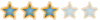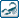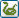yingjen likes this post

##回復： [分享]快速刪除物件(LISP)

devinchou 寫到:大家好!謝謝devinchou無私分享，有需要的朋友記得回文感謝，不要靜靜下載...靜靜離開喔😅Tiger&蘋果爸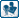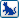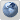##回復： [分享]快速刪除物件(LISP)asd601023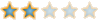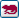##回復： [分享]快速刪除物件(LISP)ban9900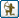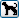##回復： [分享]快速刪除物件(LISP)

;;提點意見

`;;多圖層 / 單一圖層!!<--可以不用選;;多圖層 不是包含 單一圖層的功能嗎?;;程式的寫法;|(initget "S M") (setq L_X(getkword  (strcat "\n刪除模式[單一圖層(S)/多圖層(M)]<S>:")) ) (if (= L_X NIL)   (setq L_X "S") ) ; if.end (if (= L_X "S")   (setq LC_S 0) ) ; if.end (if (= L_X "M")   (setq LC_M 0) ) ; if.end |;;|;;通常getkword用在比2種還多的情況;;所以用getstring可簡化程式(setq LAY (getstring "\n刪除模式[多圖層 / 單一圖層<S>]:"))(if (OR (= LAY "") (= (STRCASE LAY) "S"))   (progn     (PRINT LAY)     .......   )   (progn     (PRINT "ok")     .......   ) )|;;;-----------------------------------------------------(defun C:QE_LAI (/ N LST LAY)  (setq LST "")  (setq N 0)  (while    (null      (setq SS (ssget (prompt "\n請選取要刪除物件的圖層:")))    )  )  (repeat (sslength SS)    (setq LAY (cdr (assoc 8 (entget (ssname SS N)))))    ;;(prompt (strcat "\n圖層:\n" LAY))    (setq LST (strcat LST LAY ","))    (setq N (1+ N))  )  (setq LST (substr LST 1 (1- (strlen LST)))) ;去掉最後","  (prompt (strcat "\n請選取要刪除圖層(" lst ")的物件:"))  (setq SS (ssget (list (cons 8 lst))))  (command "_.ERASE" SS ""))(princ "\nC:QE_LAI By Lucas_lai")(princ)`LUCAS_LAI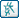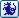##回復： [分享]快速刪除物件(LISP)nicky740821